# Capacitors in parallel

## Chapter 3 - Applications

Capacitors, like other electrical elements, can be connected to other elements either in series or in parallel. Sometimes it is useful to connect several capacitors in parallel in order to make a functional block such as the one in the figure. In such cases, it is important to know the equivalent capacitance of the parallel connection block. This article will focus on analyzing the parallel connection of capacitors and possible applications for such circuits.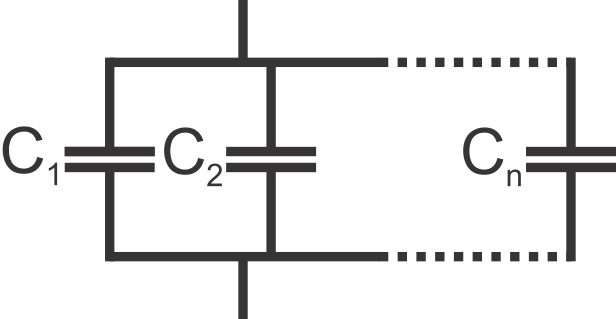## Analysis

All capacitors in the parallel connection have the same voltage across them, meaning that: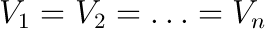where V1 to Vn represent the voltage across each respective capacitor. This voltage is equal to the voltage applied to the parallel connection of capacitors through the input wires. However, the amount of charge stored at each capacitor is not the same, and depends on the capacitance of each capacitor according to the formula: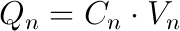where Qn is the amount of charge stored on a capacitor, Cn is the capacitance of the capacitor and Vn is the voltage applied to the capacitor, which is equal to the voltage applied to the complete parallel connection block. The total amount of charge that is stored by the block of capacitors is represented by Q and is divided between all the capacitors present in this circuit. This is represented by: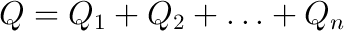The following equation is used to determine the equivalent capacitance for the parallel connection of multiple capacitors: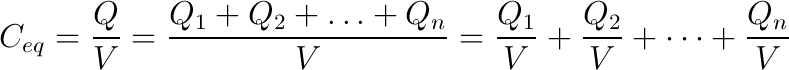where Ceq is the equivalent capacitance of the parallel connection of capacitors, V is the voltage applied to the capacitors through the input wires, and Q1 to Qn represent the charges stored at each respective capacitor. This brings us to the important conclusion that: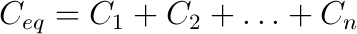which means that the equivalent capacitance of the parallel connection of capacitors is equal to the sum of the individual capacitances. This result is intuitive as well - the capacitors in parallel can be regarded as a single capacitor whose plate area is equal to the sum of plate areas of individual capacitors.

## Applications

Capacitors are devices used to store electrical energy in the form of electrical charge. By connecting several capacitors in parallel, the resulting circuit is able to store more energy since the equivalent capacitance is the sum of individual capacitances of all capacitors involved. This effect is used in some applications.

### DC power supplies

One example are DC supplies which sometimes use several parallel capacitors in order to better filter the output signal and eliminate the AC ripple. By using this approach, it is possible to use smaller capacitors that have superior ripple characteristics while obtaining higher capacitance values.

### Higher capacitance values

There are some applications which simply require capacitance values which are much higher than commercially available capacitors are able to offer. Capacitor banks are used in such applications. One example are capacitor banks used for power factor correction with inductive loads. Another example are energy storage capacitor banks which are announced for use in automotive industry, namely KERS (Kinetic Energy Recovery System) used for regenerative braking in large vehicles such as trams, as well as hybrid cars.

One high magnetic field laboratory powers the world’s most powerful magnet capable of delivering a magnetic field of almost 100 teslas by storing energy in a capacitor bank. The stored energy is released through a magnetic coil in a very short amount of time, generating a very powerful magnetic field.

In any case, capacitor banks can reach very high capacitance values. By using several supercapacitors connected in parallel, capacitances of several tens of kilofarads are feasible, especially bearing in mind that supercapacitors are capable of achieving capacitance values of over 2000 farads.

## Design restrictions

When connecting capacitors in parallel, there are some points to keep in mind. One is that the maximum rated voltage of a parallel connection of capacitors is only as high as the lowest voltage rating of all the capacitors used in the system. Thus, if several capacitors rated at 500V are connected in parallel to a capacitor rated at 100V, the maximum voltage rating of the complete system is only 100V, since the same voltage is applied to all capacitors in the parallel circuit.

## Safety

Another point to keep in mind is that capacitor banks can be dangerous due to the amount of energy stored and the fact that capacitors are able to release the stored energy in a very short amount of time. This stored energy is sometimes efficient to cause serious injuries or damage to electrical wiring and devices if shorted out by accident.